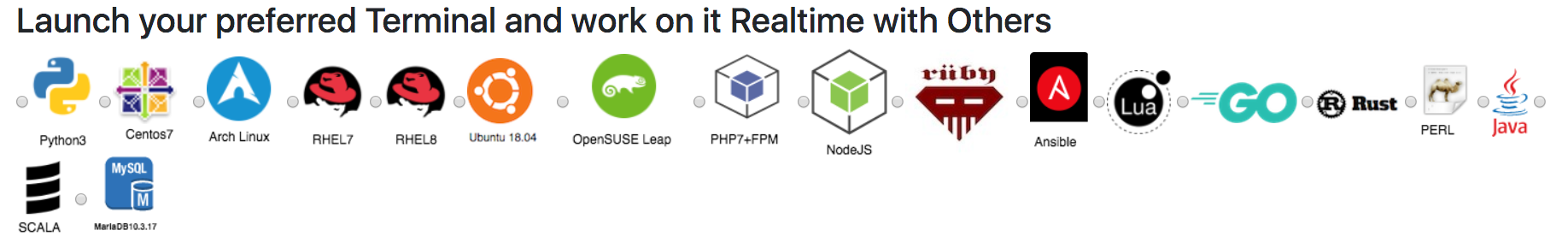# Diffie-Hellman Key Exchange

Diffie-Hellman Key Exchange, The protocol allows two users to exchange a secret key over an insecure medium without any prior secrets,The Setup Suppose we have two people wishing to communicate: Alice and BobThey do not want Eve (eavesdropper) to know their message.Alice and Bob agree upon and make public two numbers g and p, where p is a prime and g is a primitive root mod p

DH Parameter G :
DH Parameter P :

Thanks for using this software, for Cofee/Beer/Amazon bill and further development of this project please Share.

Any private key value that you enter or we generate is not stored on this site, this tool is provided via an HTTPS URL to ensure that private keys cannot be stolen, for extra security run this software on your network, no cloud dependency

Asking for donation sound bad to me, so i'm raising fund from by offering all my eight book for just \$9## Diffie Helman Key Exchange

Source https://security.stackexchange.com/questions/45963/diffie-hellman-key-exchange-in-plain-english
Diffie-Hellman is an algorithm used to establish a shared secret between two parties. It is primarily used as a method of exchanging cryptography keys for use in symmetric encryption algorithms like AES.
The algorithm in itself is very simple. Let’s assume that Alice wants to establish a shared secret with Bob.
Alice and Bob agree on a prime number, p, and a base, g, in advance. For our example, let’s assume that p=23 and g=5.
Alice chooses a secret integer a whose value is 6 and computes A = g^a mod p. In this example, A has the value of 8.
Bob chooses a secret integer b whose value is 15 and computes B = g^b mod p. In this example, B has the value of 19.
Alice sends A to Bob and Bob sends B to Alice.
To obtain the shared secret, Alice computes s = B^a mod p. In this example, Alice obtains the value of s=2
To obtain the shared secret, Bob computes s = A^b mod p. In this example, Bob obtains the value of s=2.
The algorithm is secure because the values of a and b, which are required to derive s are not transmitted across the wire at all.##### Online Terminals